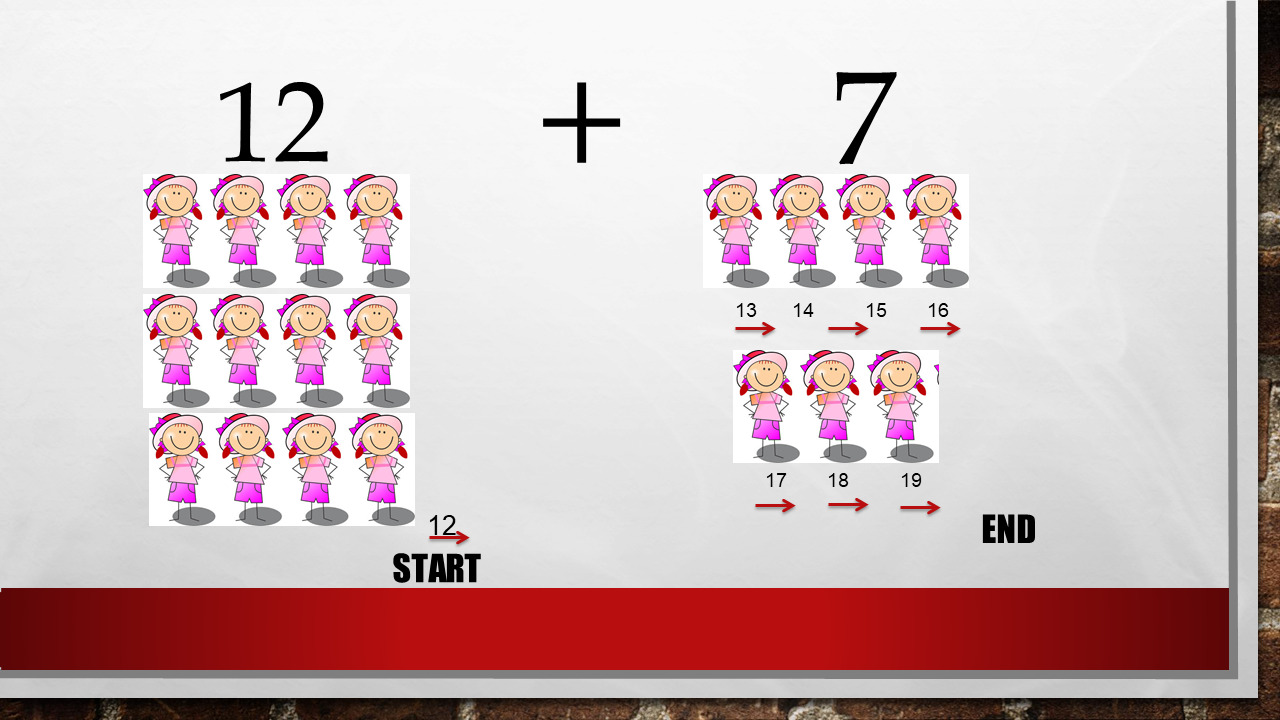1
visibility

Find the sum of the given numbers: 7 + 12

• A

19

• B

18

• C

20

• D

21

The question is exactly 12 + 7 = ?

Now, we can count from any number. Count on!

So, 12 + 7 = ?

We count  7 numbers from 12.

From 12, 13, 14, 15, 16, 17, 18, 19

So: 7 + 12 = 19